1

# Machine Learning for Design

Lecture 8

Design and Develop Machine Learning Models - Part 2

2

## ML Algorithms on Structured Data

3

### Decision Trees

• Trained with labelled data (supervised learning)
• classes —> classification
• values —> regression
• Simple model that resembles human reasoning:
• Answering a lot of yes/no questions based on feature values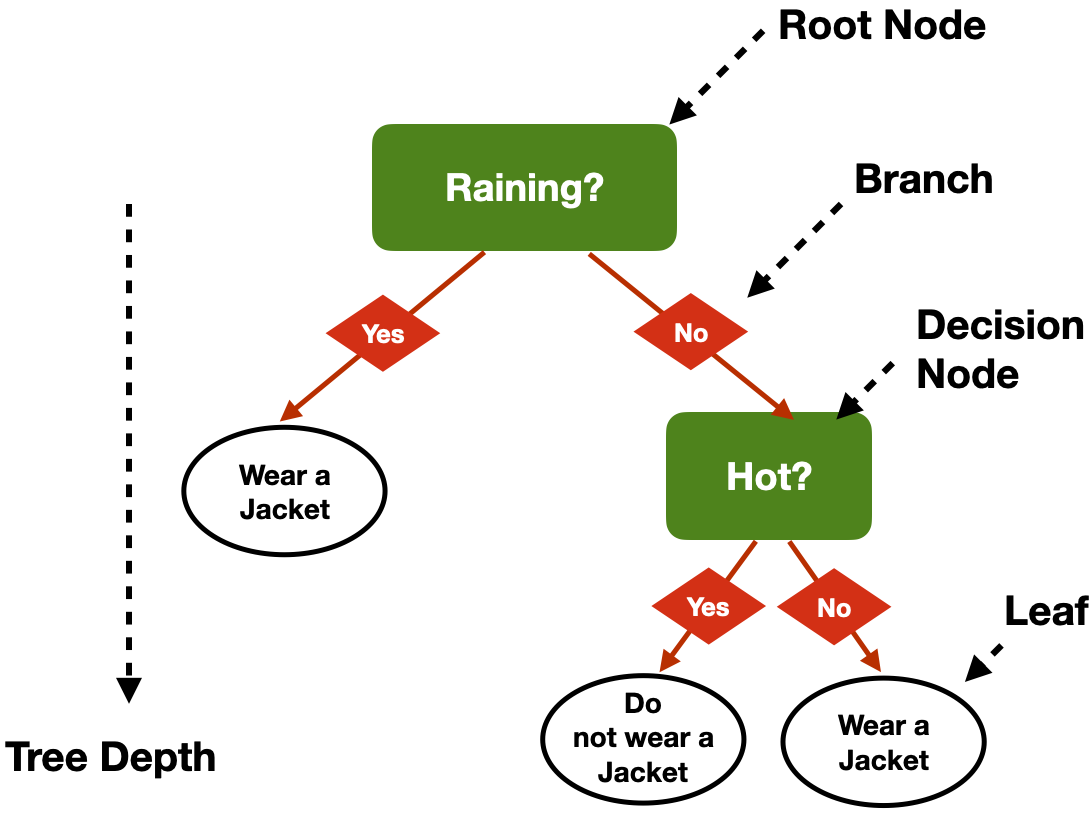4

#### Problems

• How many questions? (Tree depth)
• In which order?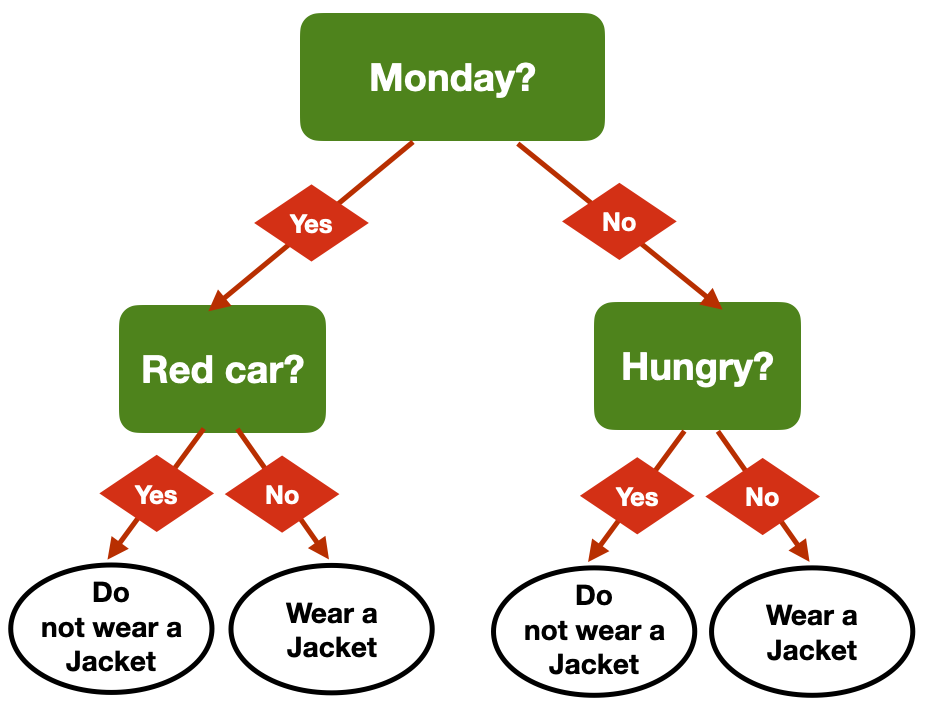5

#### Same Problem, Multiple Trees

• Am I hungry?
• Is there a red car outside?
• Is it Monday?
• Is it raining?
• Is it cold outside?6

#### Same Problem, Multiple Trees

• Am I hungry?
• Is there a red car outside?
• Is it Monday?
• Is it raining?
• Is it cold outside?7

#### Same Problem, Multiple Trees

• Am I hungry?
• Is there a red car outside?
• Is it Monday?
• Is it raining?
• Is it cold outside?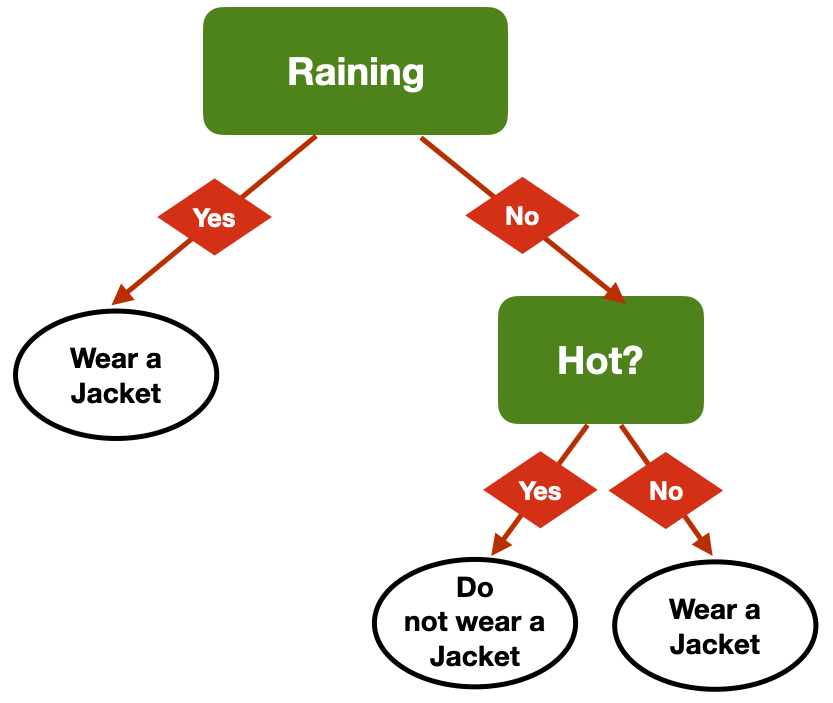8

#### Same Decision, different tress9

#### How to decide the best question to ask?

• Accuracy
• Which question helps me be correct more often?
• Gini Impurity Index
• A measure of diversity in a dataset —> diversity of classes in a given leaf node
• index = 0 means that all the items in a leaf node have the same class
• Which question helps me obtain the lowest average Gini impurity Index?
• Entropy
• Another measure of diversity linked to information theory
• Which question helps me obtain the lowest average entropy?
10

#### Building the tree (pseudo-code)

• Add a root node, and associate it with the entire dataset
• This node has level 0. Call it a leaf node
• Repeat until the stopping conditions are met at every leaf node
• Pick one of the leaf nodes at the highest level
• Go through all the features, and select the one that splits the samples corresponding to that node in an optimal way, according to the selected metric.
• Associate that feature to the node
• This feature splits the dataset into two branches
• Create two new leaf nodes, one for each branch
• Associate the corresponding samples to each of the nodes
• If the stopping conditions allow a split, turn the node into a decision node, and add two new leaf nodes underneath it
• If the level of the node is $i$, the two new leaf nodes are at level $i + 1$
• If the stopping conditions don’t allow a split, the node becomes a leaf node
• Associate the most common label among its samples
• That label is the prediction at the leaf
11

#### A geometrical perspective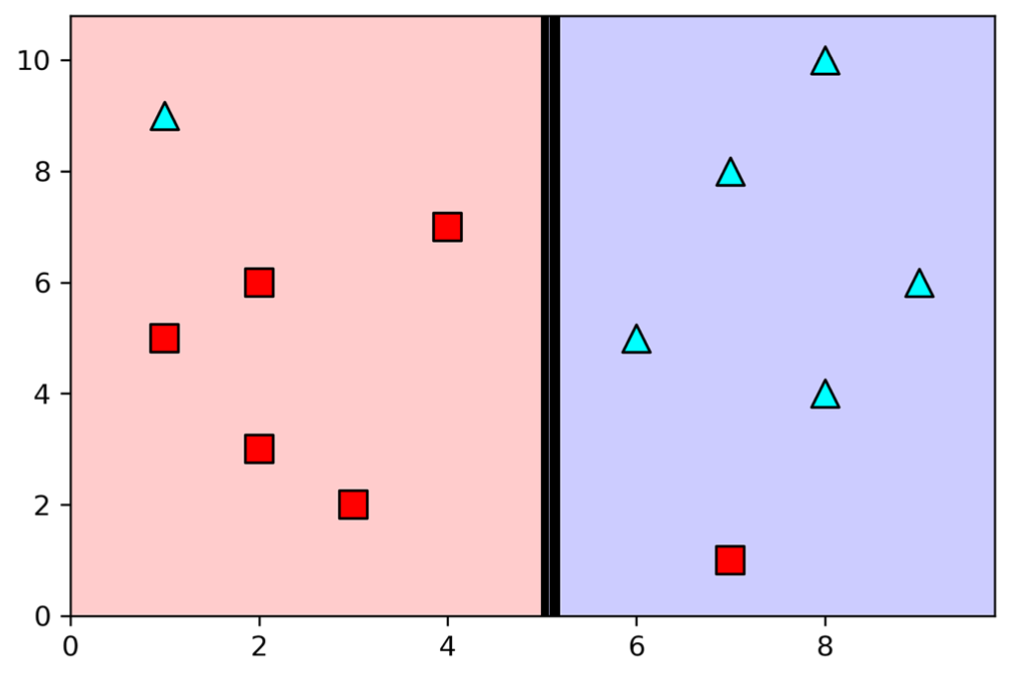• Step 1 - Select the first question
• $X>=5$
• Best possible prediction accuracy with one feature
12

#### A geometrical perspective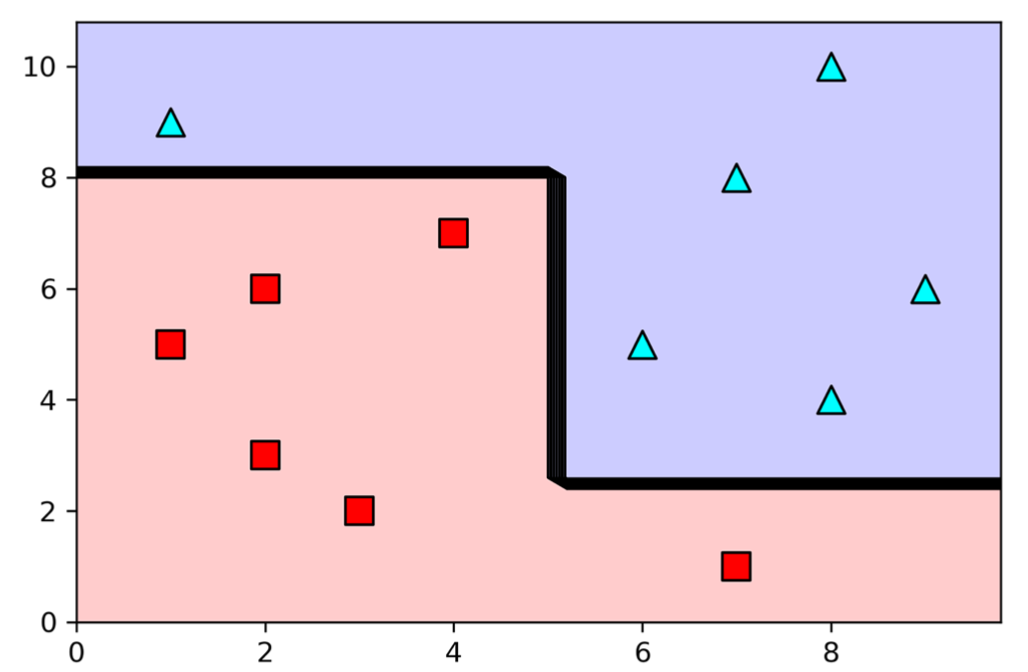• Step 2 - Iterate
• $x<5 \& y<8$;
• $x>=5 \& y>=2$
• Perfect split of the feature space
13

#### Decision Trees: Pros

• Simple to understand and interpret.
• Trees can be visualized
• Requires little data preparation
• Other techniques often require data normalisation, dummy variables need to be created, and blank values need to be removed
• Able to handle both numerical and categorical data
14

#### Decision Trees: Cons

• Possible to create over-complex trees that do not generalize the data well
• overfitting
• Unstable —> small variations in the data might result in a completely different tree being generated
• Biased trees if some classes dominate
15

### Ensemble Learning

Idea: combine several “weak” learners to build a strong learner

Random Forest: Weak learners are decision trees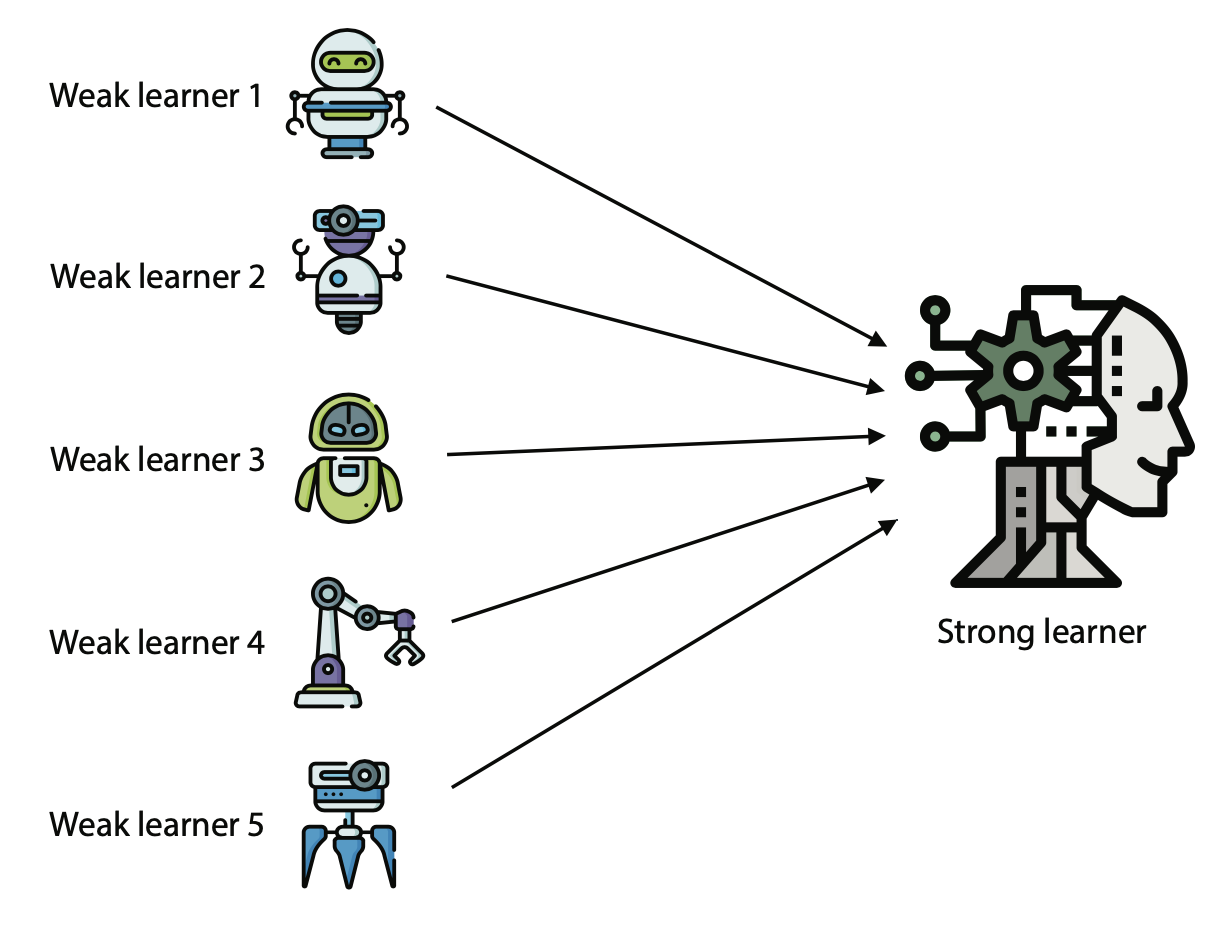16
• Build random training sets from the dataset
• Train a different model on each of the sets
• weak learners
• Combination the weak models by voting (if it is a classification model) or averaging the predictions (if it is a regression model)
• For any input, each of the weak learners predicts a value
• The most common output (or the average) is the output of the strong learner
17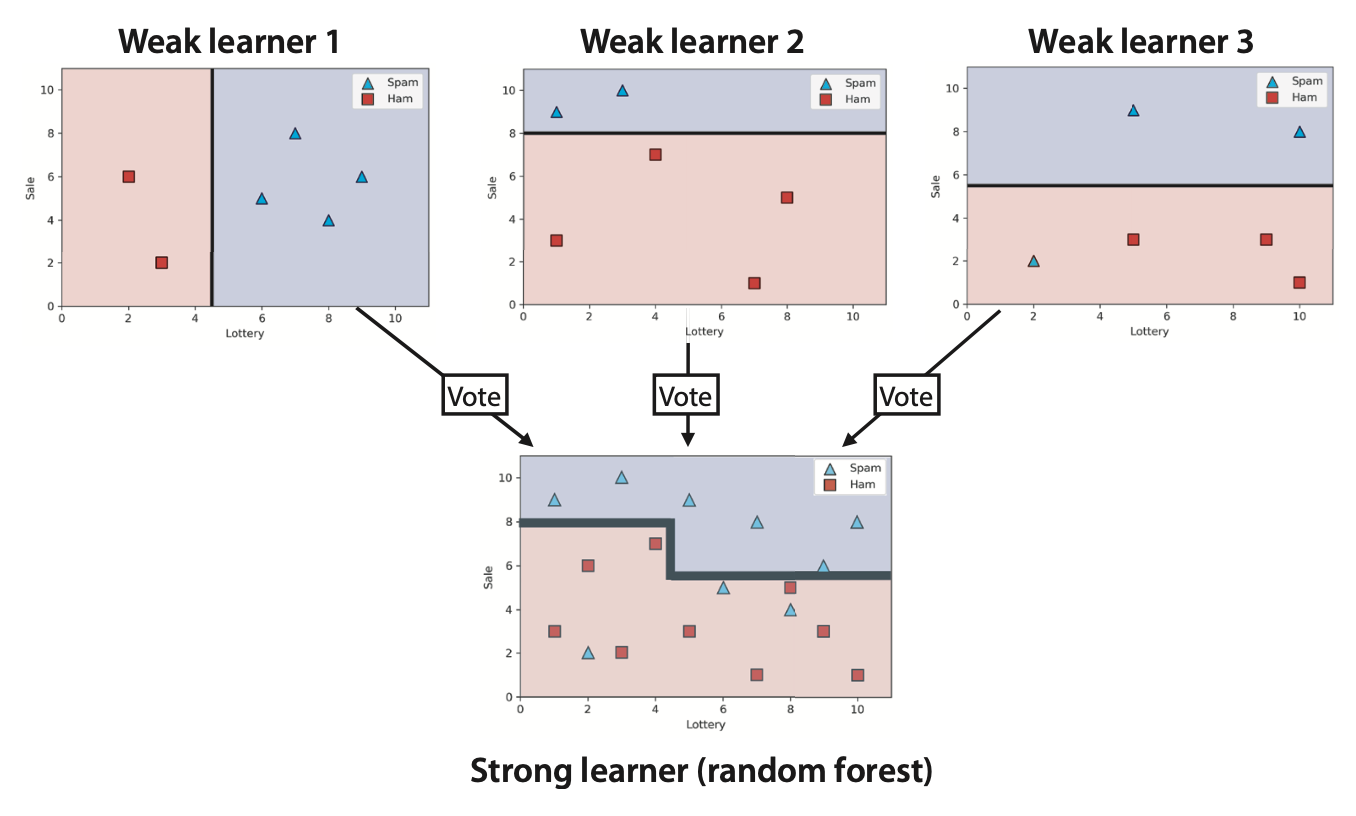18

### Clustering

19

#### What is clustering?

• Grouping items that “belong together” (i.e. have similar features)
• Unsupervised learning: we only use data features, not the labels\u2028
20
• We can detect patterns
• Group emails or search results
• Customer shopping patterns
• Regions of images
• Useful when you don’t know what you’re looking for
• But: can give you gibberish
• If the goal is classification, we can later ask a human to label each group (cluster)
21

#### Why do we cluster?

• Summarizing data
• Look at large amounts of data
• Represent a large continuous vector with the cluster number
• Counting
• Computing feature histograms
• Prediction
• Images in the same cluster may have the same labels
• Segmentation
• Separate the image into different regions
22

#### K-Means

• An iterative clustering algorithm
• Initialize: Pick K random points as cluster centres
• Alternate:
• Assign data points to the closest cluster centre
• Change the cluster centre to the average of its assigned points
• Stop when no points’ assignments change
23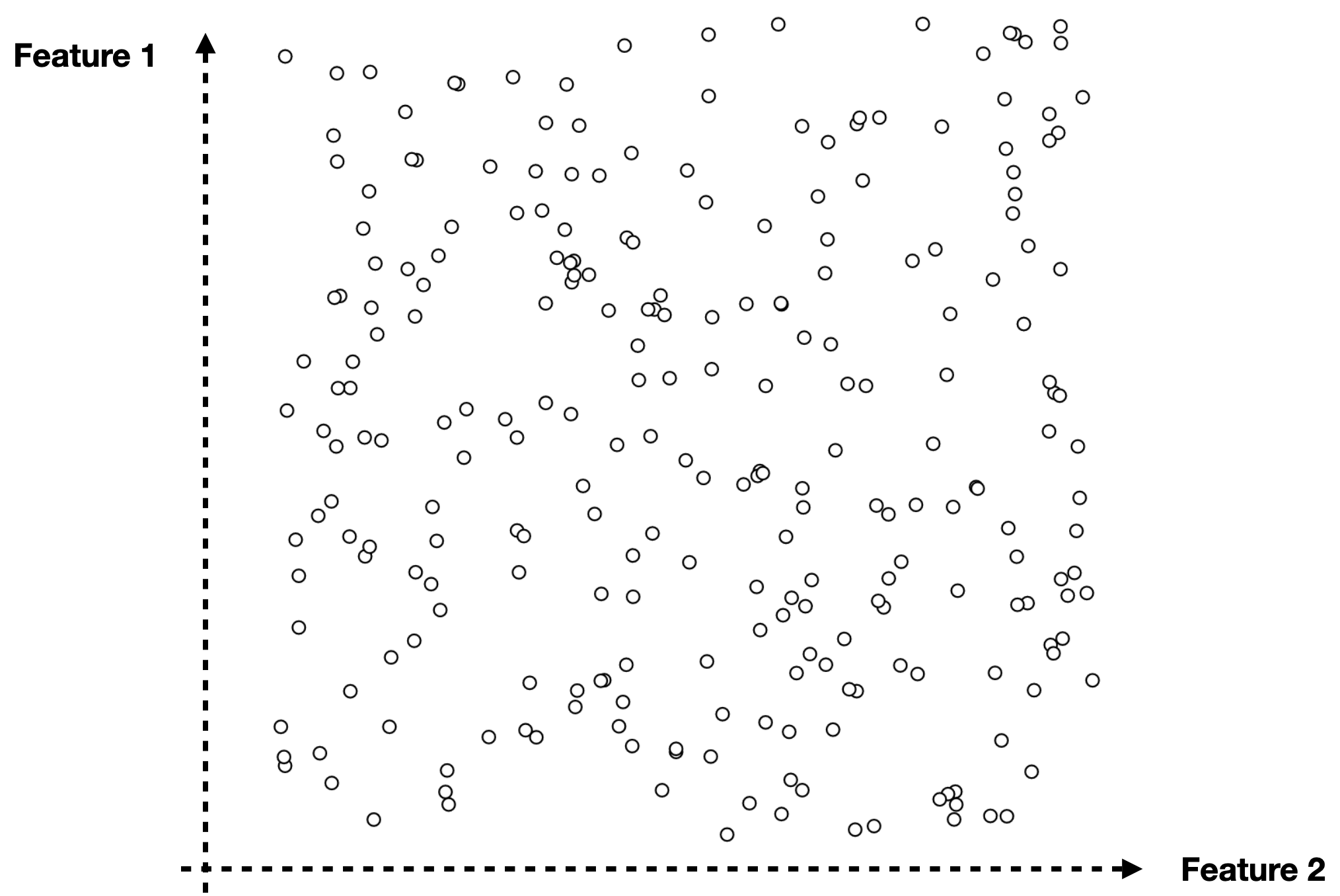24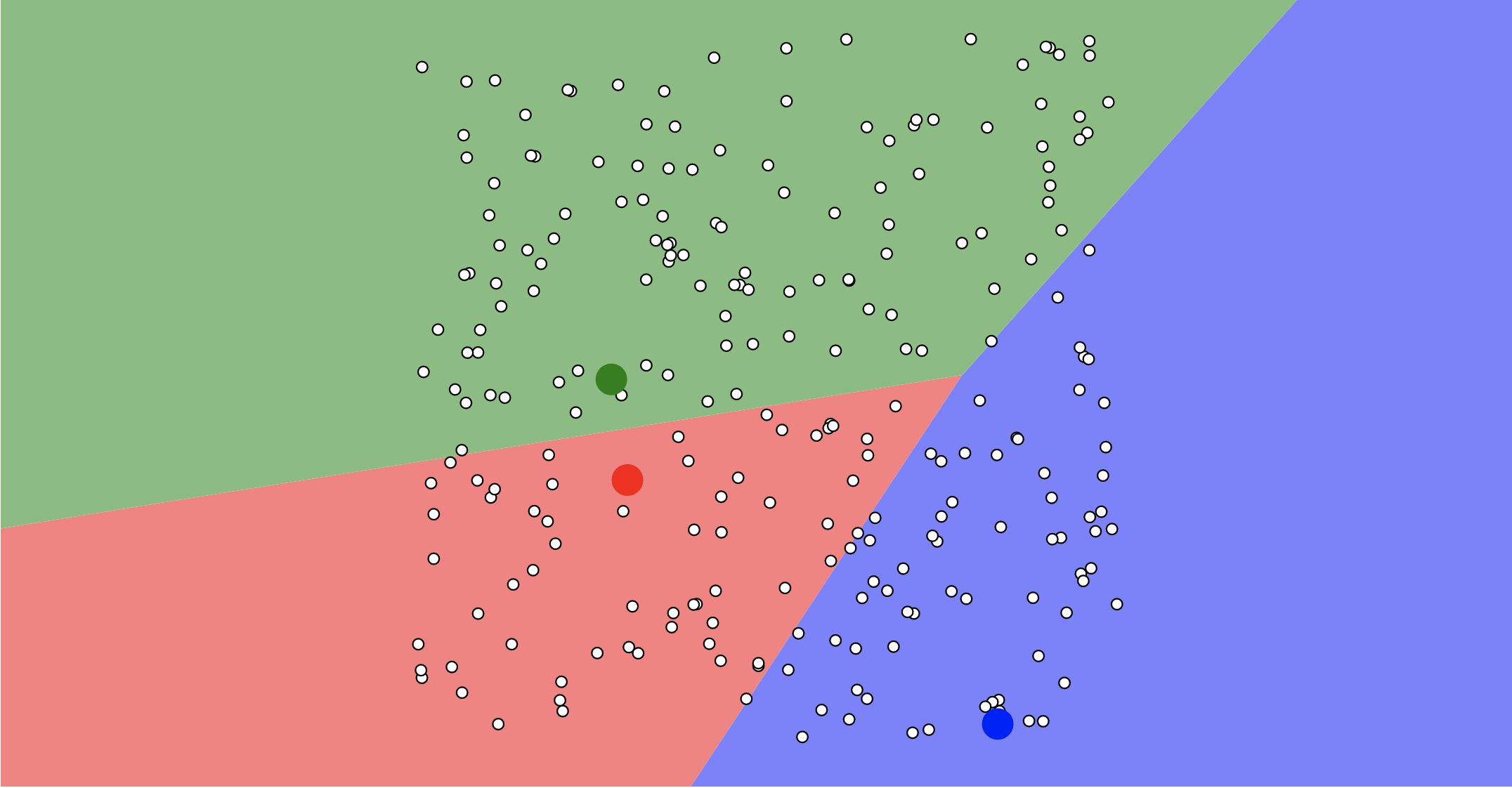25
##### Assign Data Points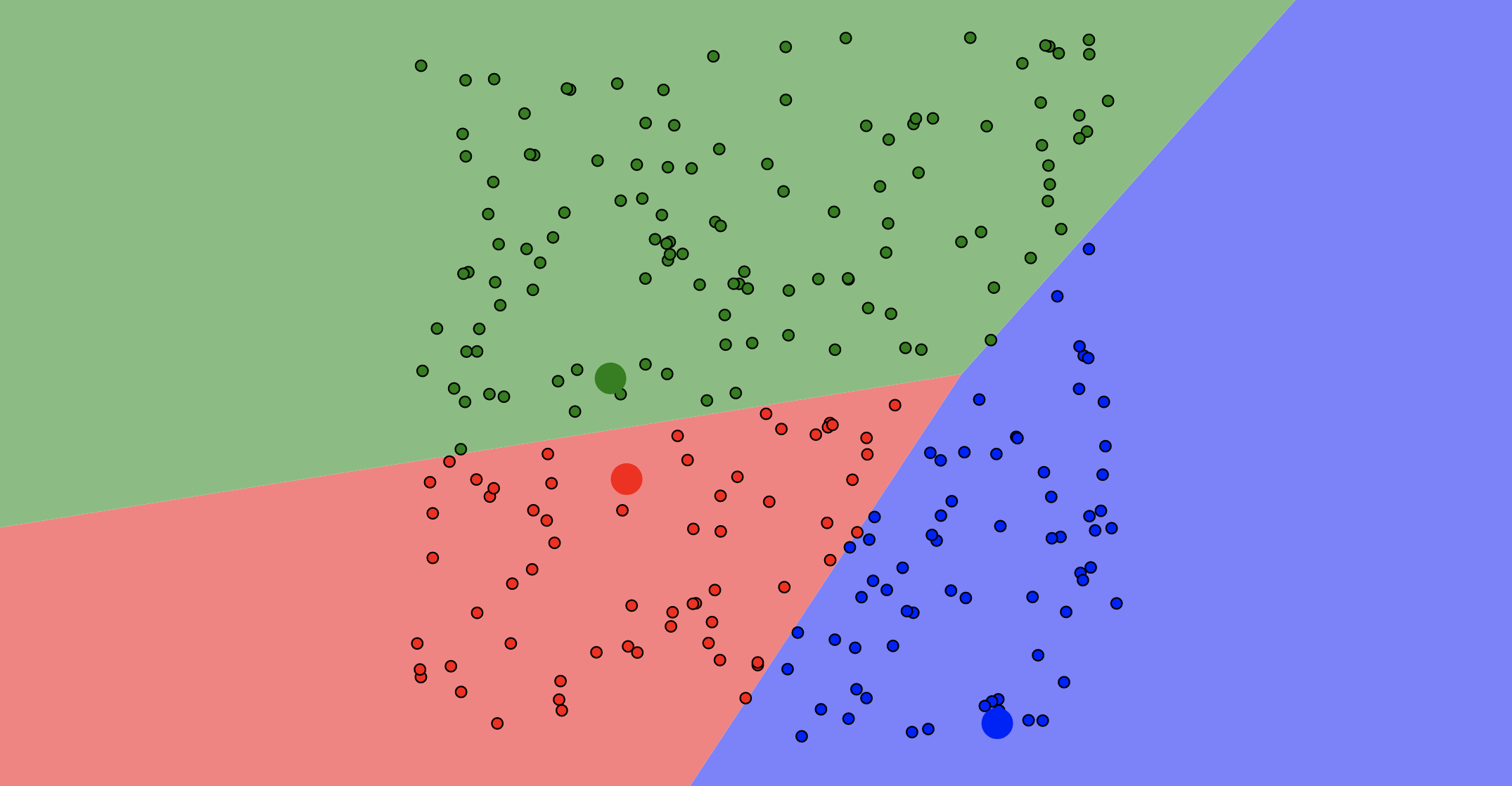26
##### Update Centroids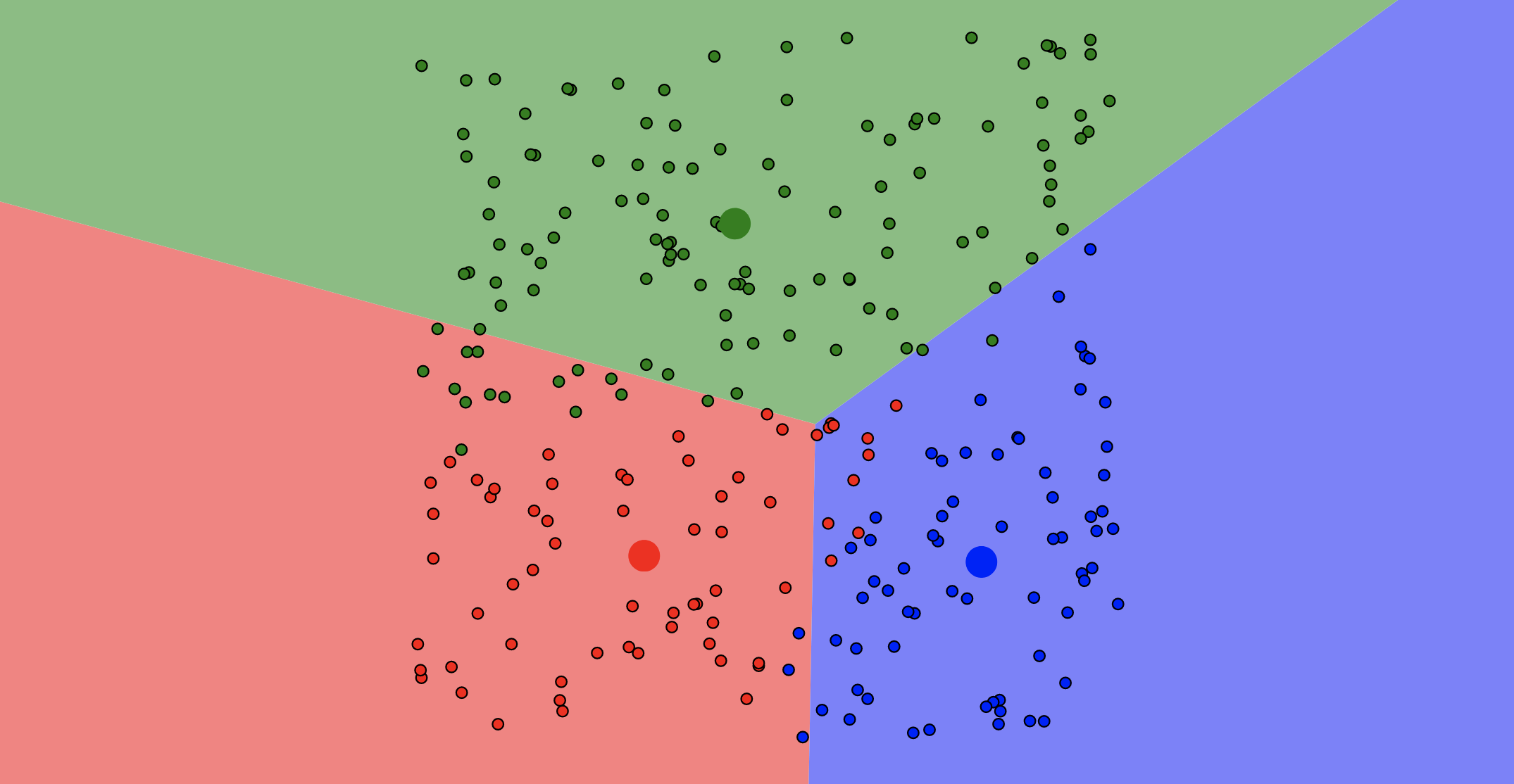27
##### Re-Assign Data Points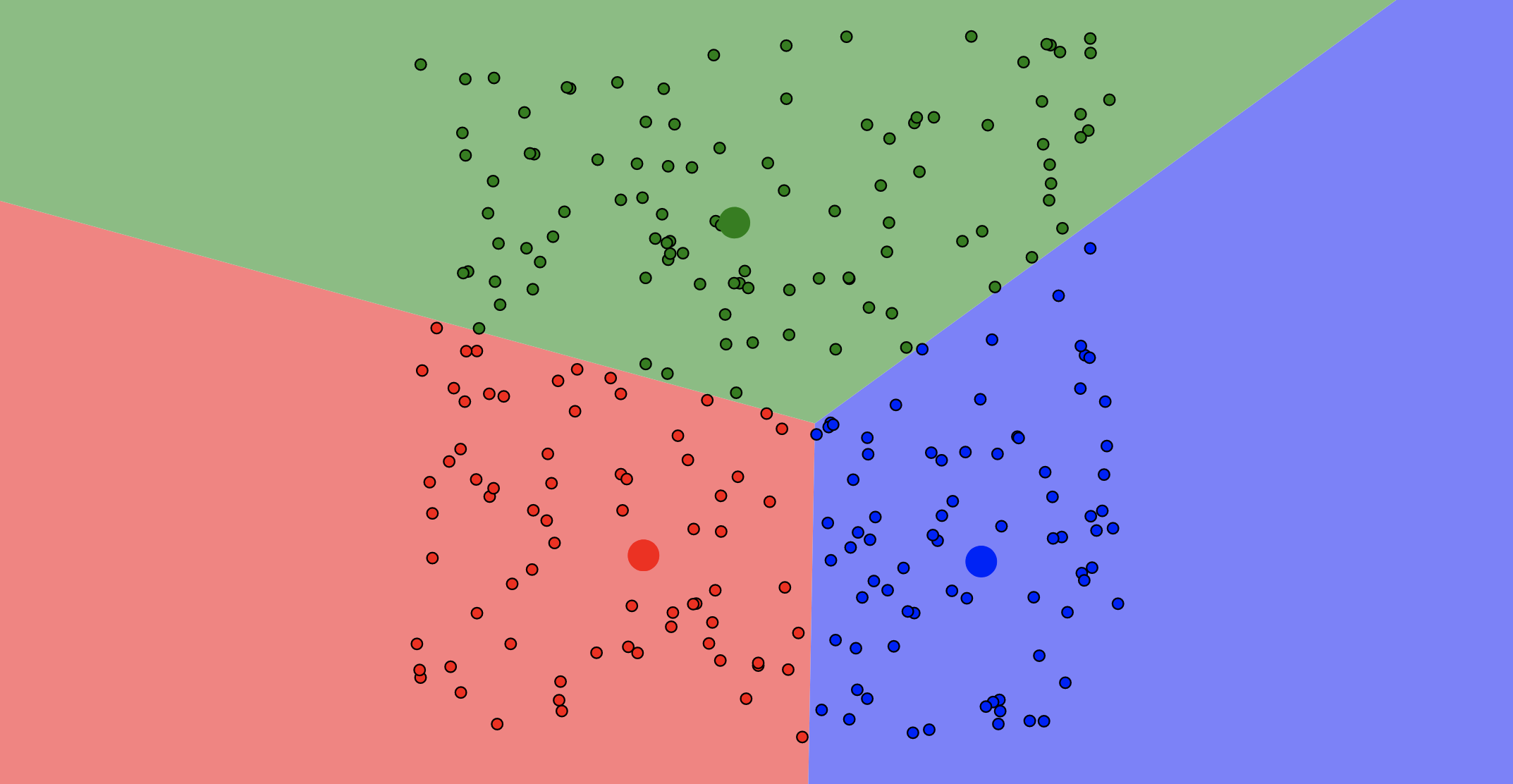28
##### Update Centroids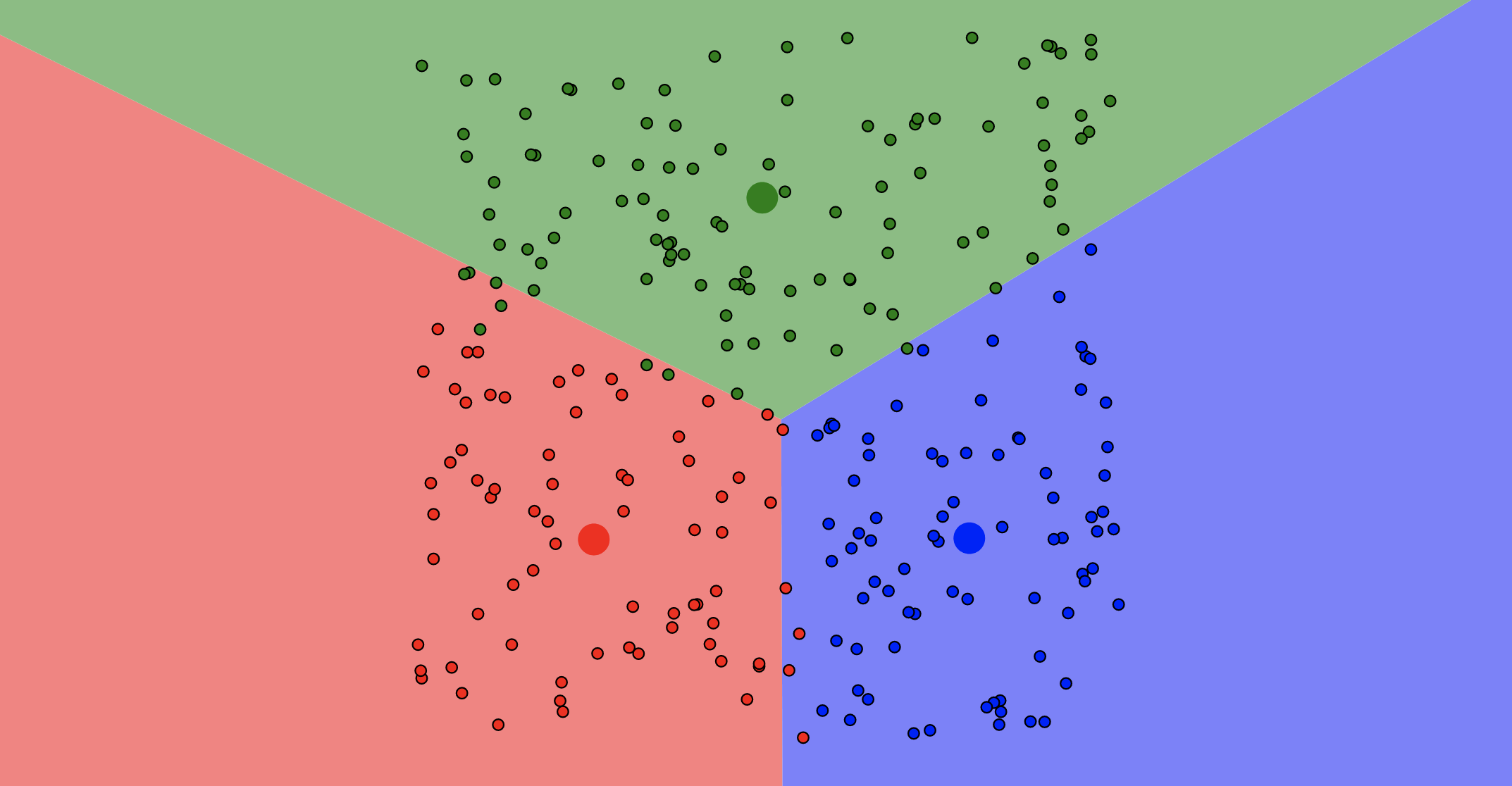29
##### Re-Assign Data Points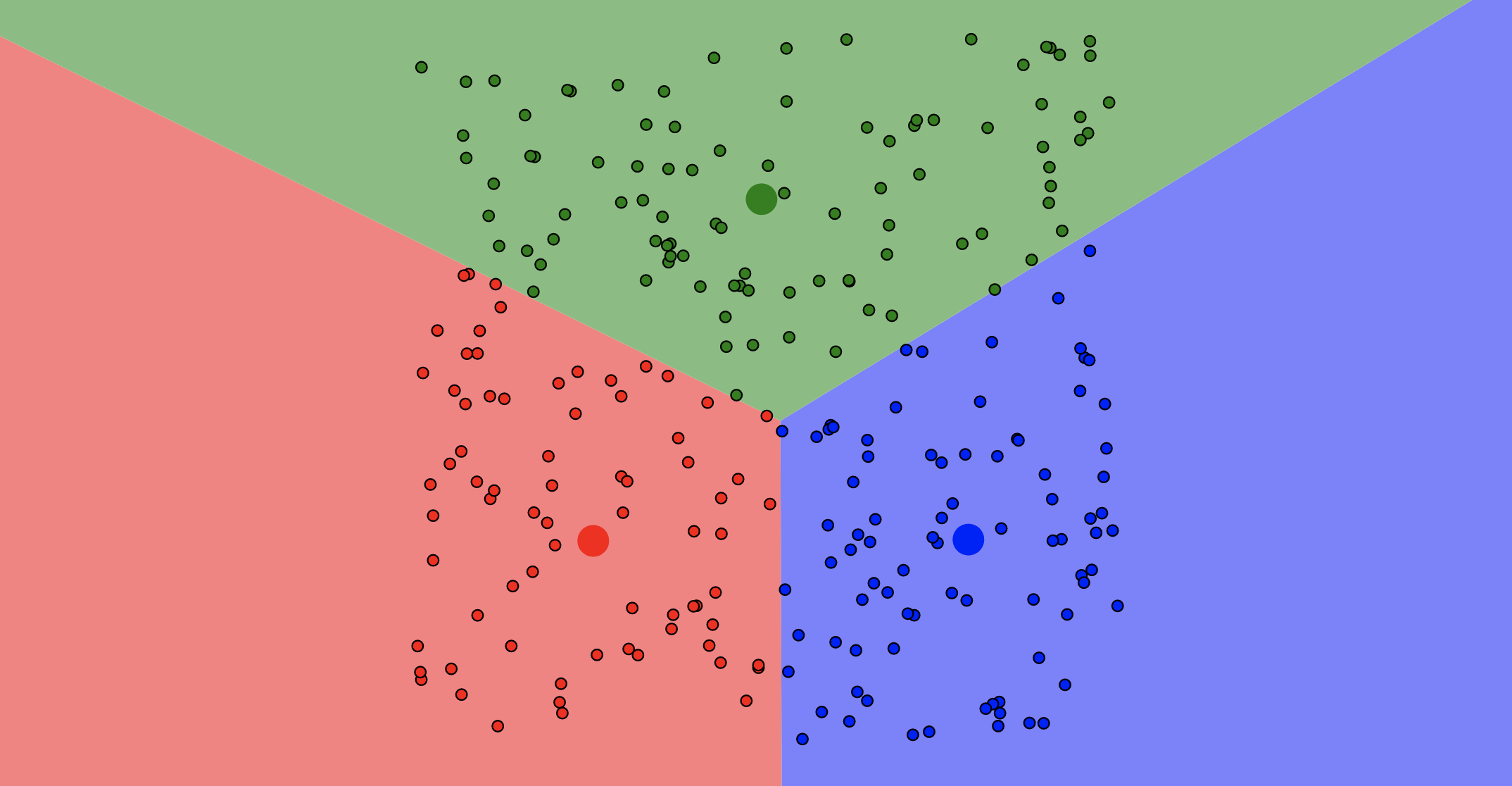30
##### Update Centroids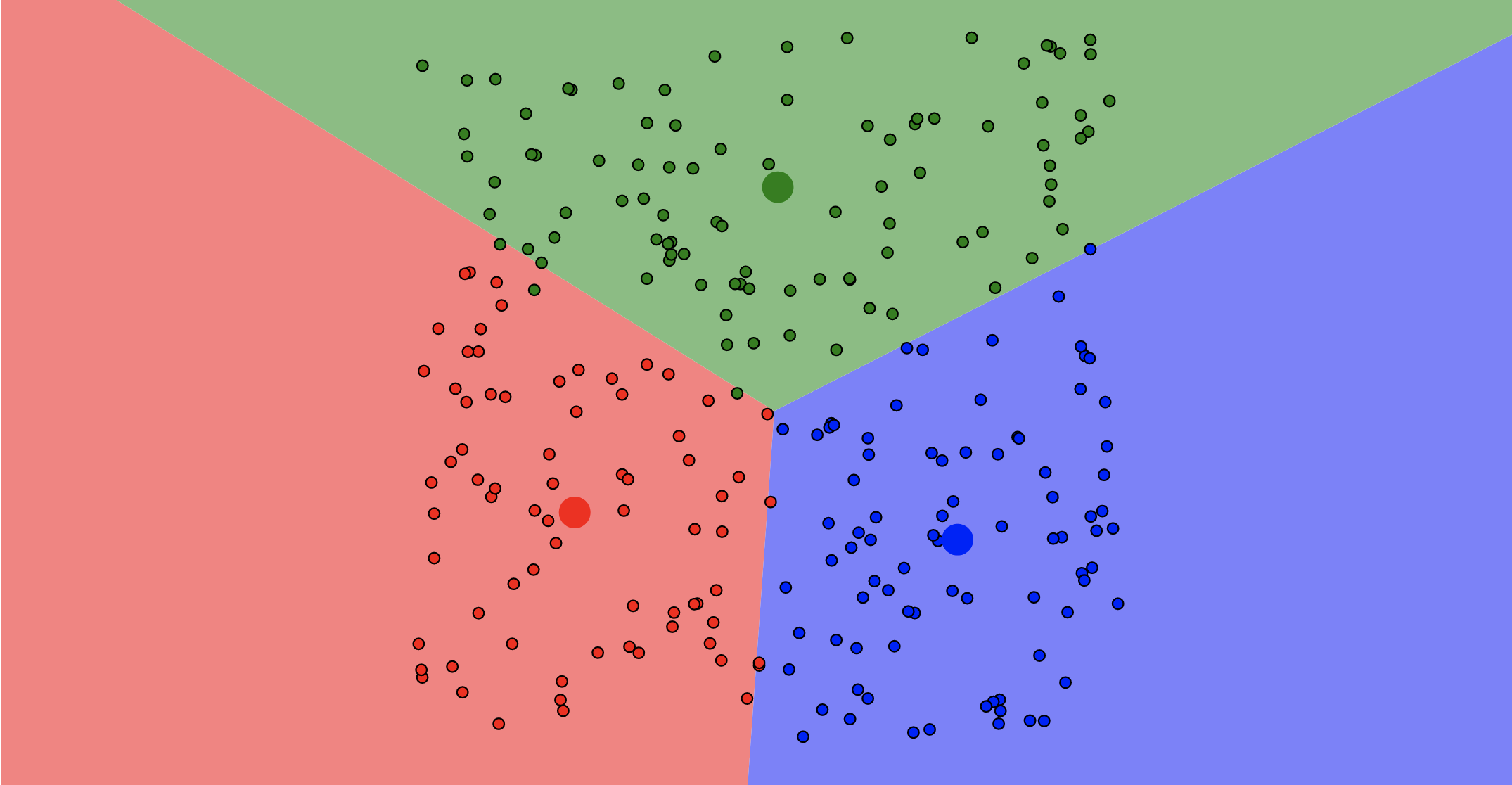31
##### Re-Assign Data Points - Stop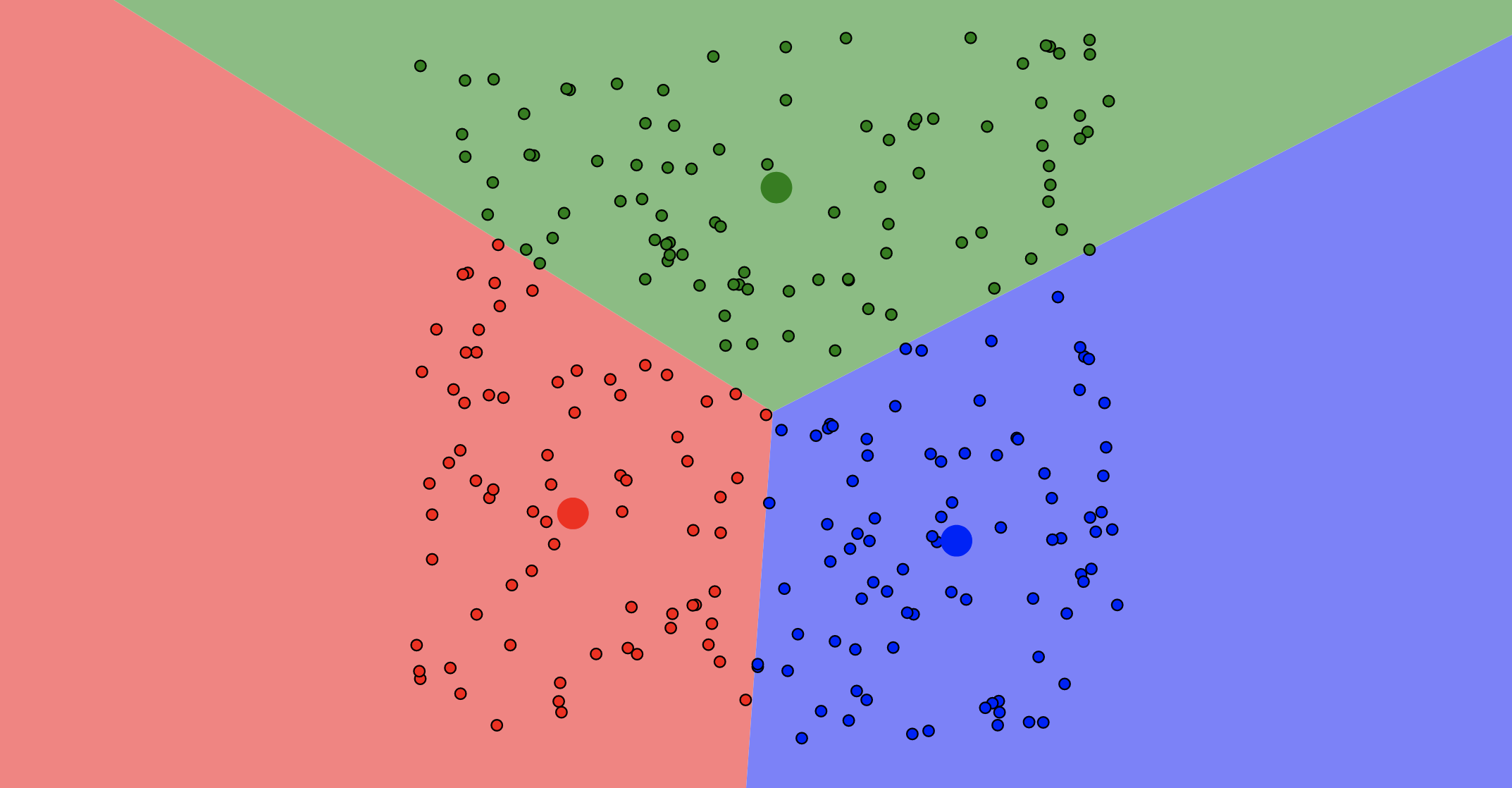32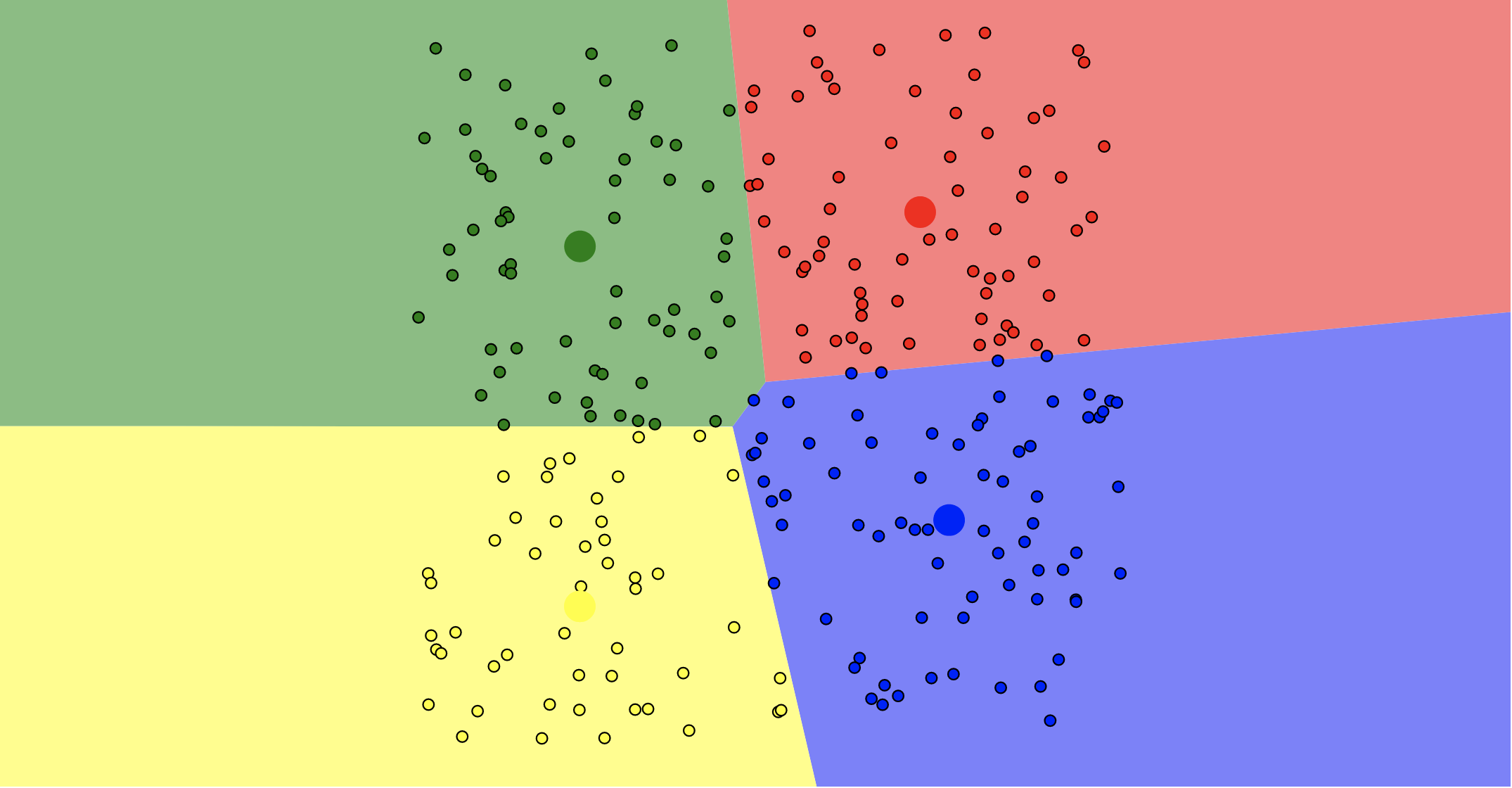33

#### K-Means Pros

• Simple, fast to compute
• Guaranteed to converge in a finite number of iterations
34

#### K-Means Cons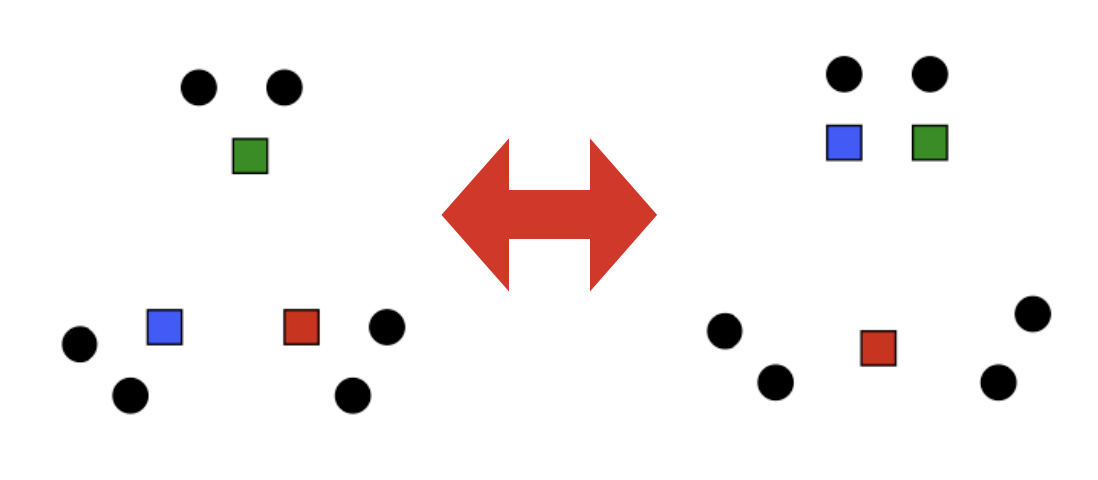• Setting k?
• One way: silhouette coefficient
• Algorithm is heuristic
• It does matter what random points you pick!
• Sensitive to outliers
35

#### Example of K-means not working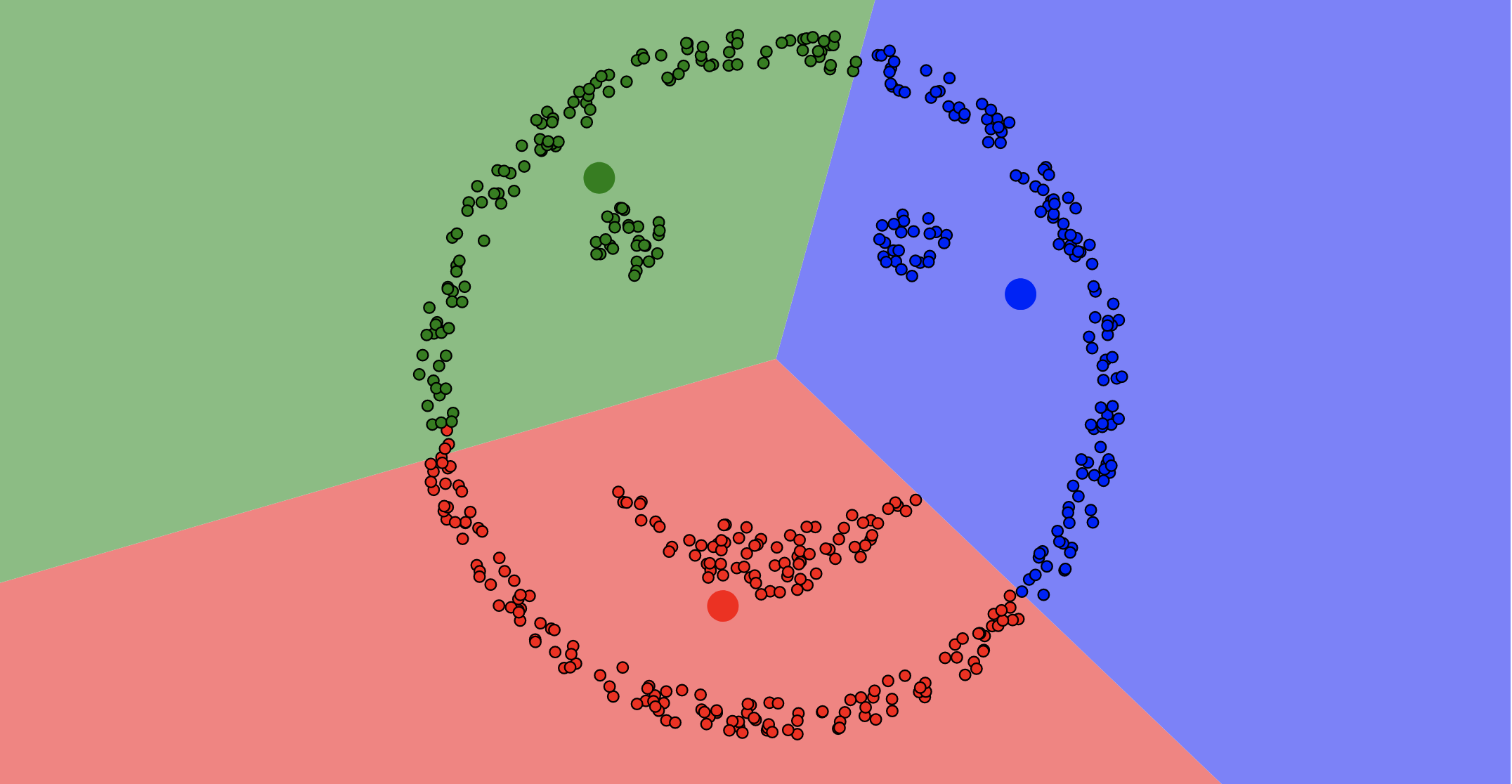36

## Back to Evaluation

37
• No free-lunch: there is no one best machine learning algorithm for all problems and datasets
• How well does a learned model generalize to a new evaluation set?
• Challenge: achieving good generalization and a small error
38

### Underfitting vs. Overfitting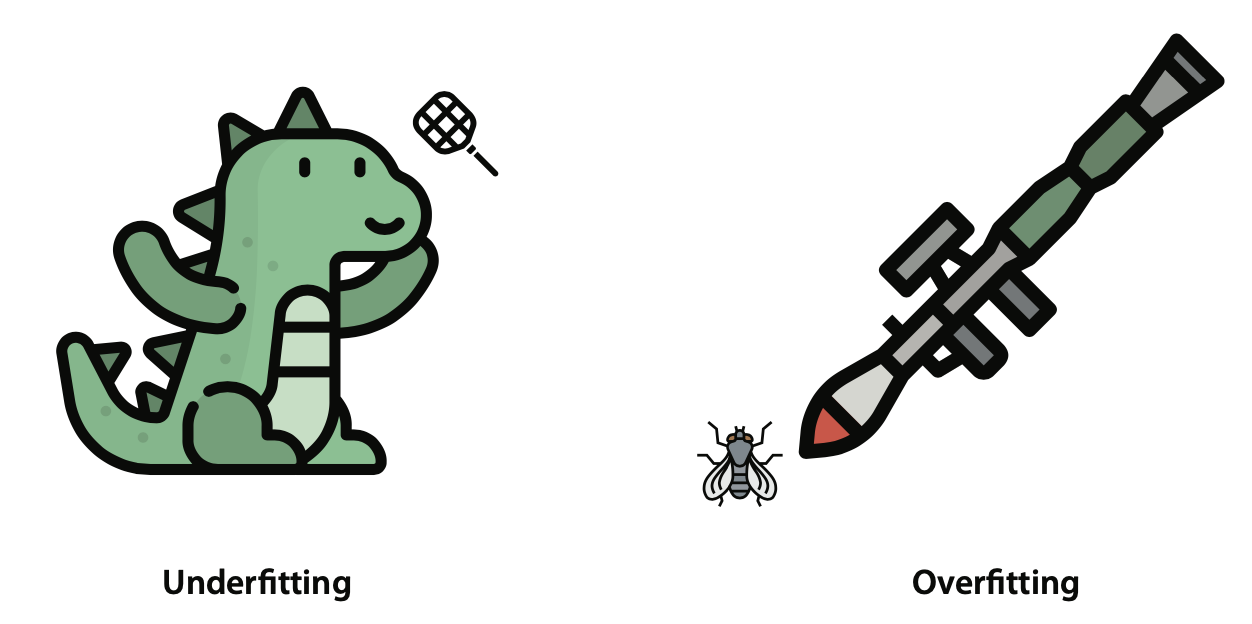39

### Regression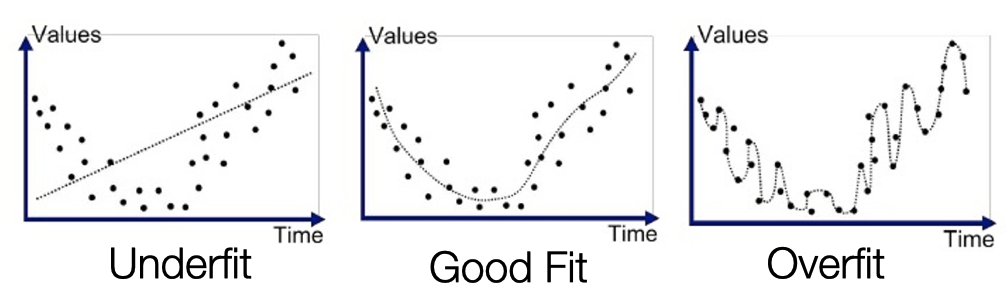40

### Classification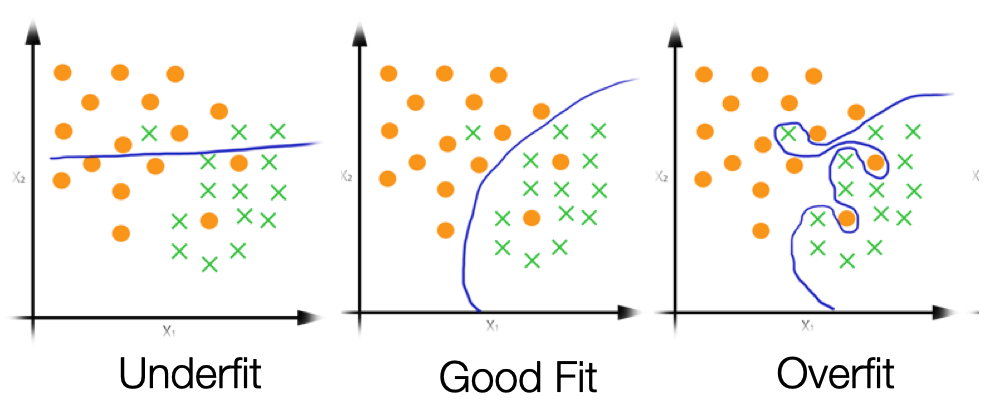41

### Components of expected loss

• Noise in data: unavoidable
• Bias: how much the average model differs from the true model
• Error due to inaccurate assumptions/simplifications made by the model
• Variance: how much models estimated from different training sets differ from each other
• Too much sensitivity to the samples
42
43
44

### Protect Against overfitting

Low bias and high variance

Low training error and high test error

• The model
• is too complex
• matches too closely the idiosyncrasies (noise) of the training data
45

### Protect Against underfitting

High bias and low variance

High training error and high test error

• The model
• is too simple
• does not adequately capture the patterns in the training data
46
47

### Tuning Hyper-parameters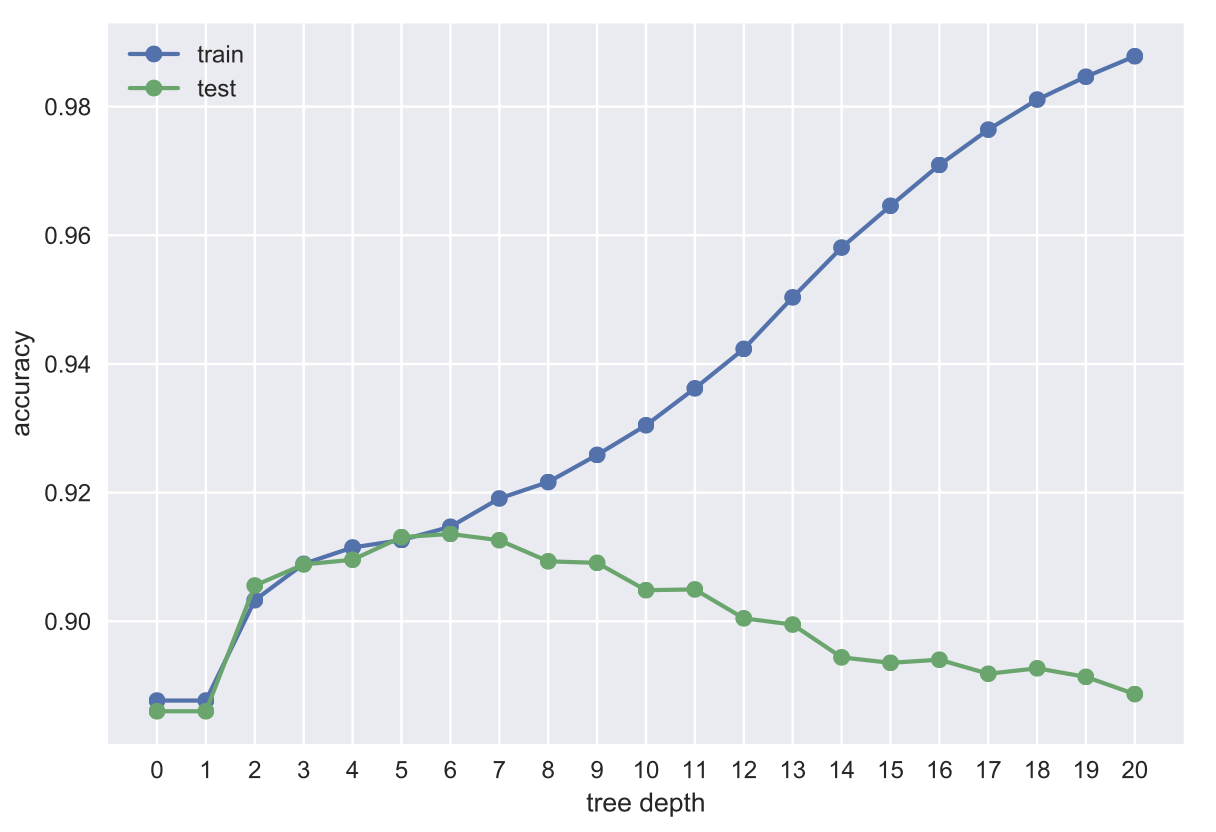• Hyper-parameter: Inputs to the learning algorithms that control their behavior
• Examples:
• maximum tree depth in decision trees
• number of neighbors $k$ in k-nearest neighbor
• Neural networks: architecture, learning rate
48

### Tuning Hyper-parameters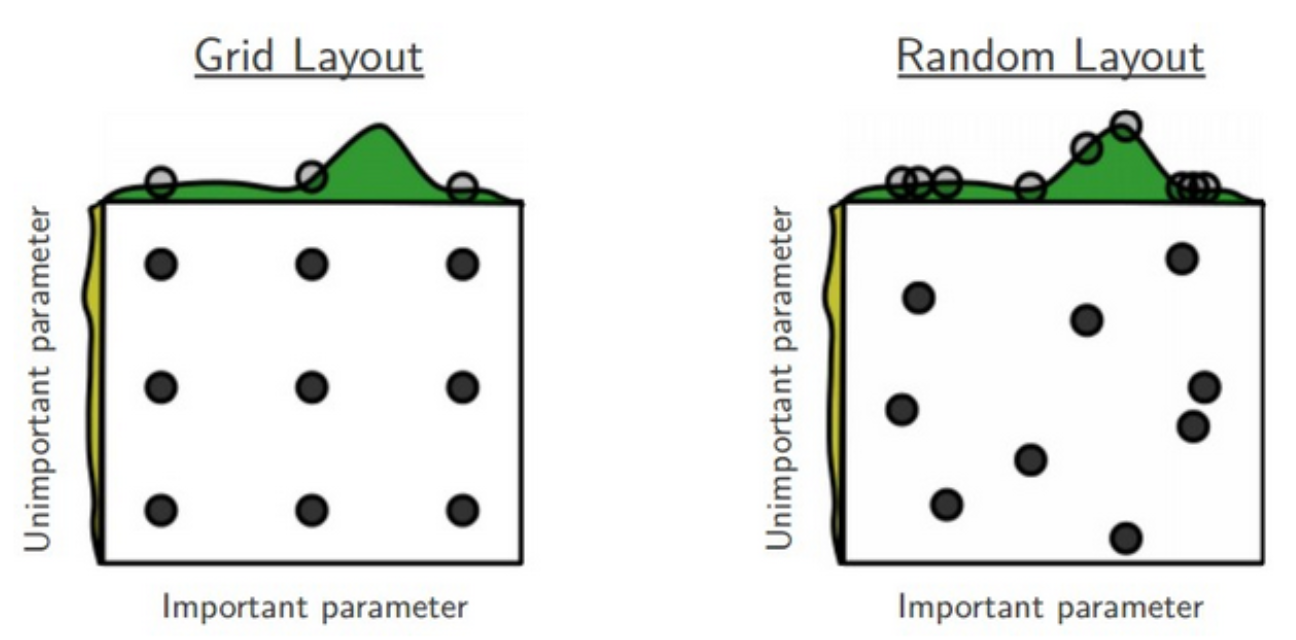• For a model to work well, they often need to be tuned carefully
• Huge search space! may be inefficient to search exhaustively
49

### Tuning Hyper-parameters: Approaches• DON’T optimise these numbers by looking at the test set!That is CHEATING!
• Grid search: brute-force exhaustive search among a finite set of hyper-parameter settings
• All combinations are tried, then the best setting selected
• Random search: for each hyper-parameter, define a distribution (e.g., normal, uniform)
• In the search loop, we sample randomly from these distributions
50

### Double Cross-Validation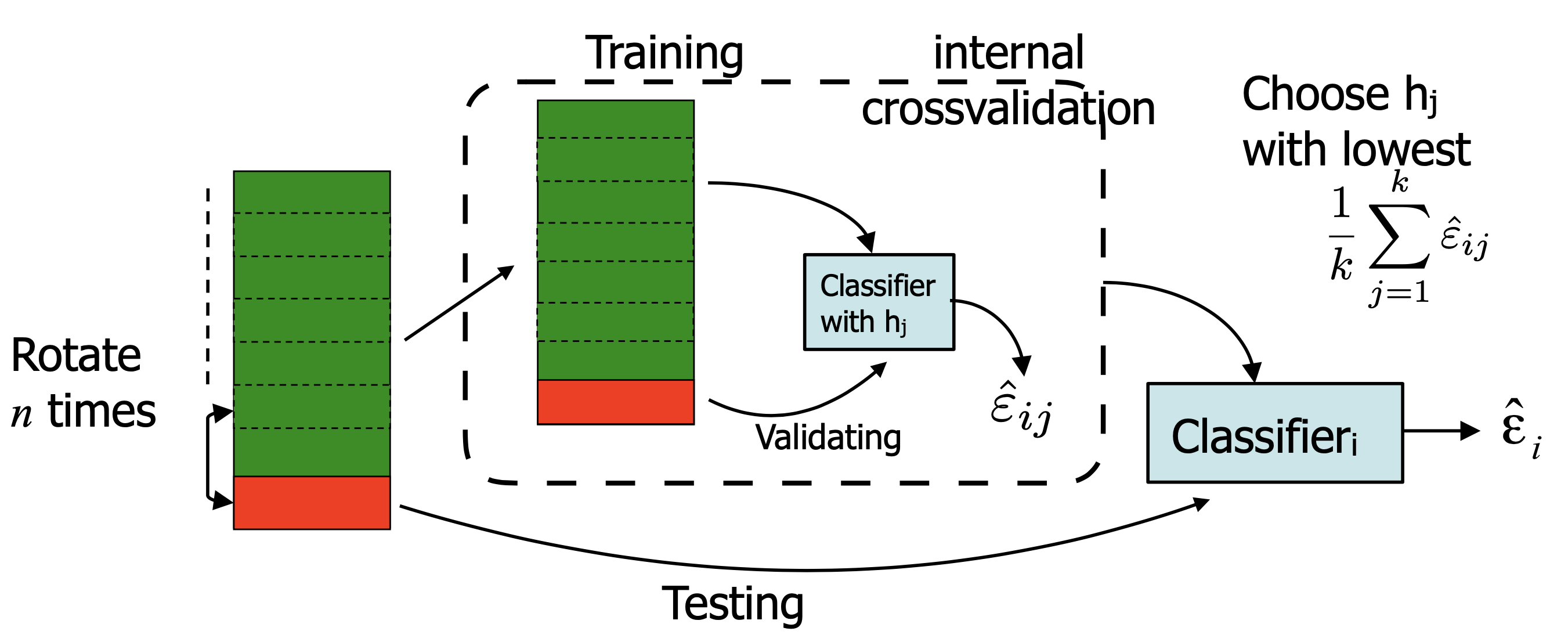• Cross-validation inside another cross-validation
• To optimise over the hyperparameter
• The minimum error is often not the most interesting. Try to understand the advantages/disadvantages
• What errors are made? (inspect objects, inspect labels)
• What classes are problematic? (confusion matrix)
• Does adding training data help? (learning curve)
• How robust is the model?
51

# Machine Learning for Design

Lecture 8

Design and Develop Machine Learning Models - Part 2

52

#### Credits

Grokking Machine Learning. Luis G. Serrano. Manning, 2021

[CIS 419/519 Applied Machine Learning]. Eric Eaton, Dinesh Jayaraman.

https://scikit-learn.org/stable/modules/tree.html

Deep Learning Patterns and Practices - Andrew Ferlitsch, Maanning, 2021

Machine Learning Design Patterns - Lakshmanan, Robinson, Munn, 2020